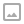## 「ZJOI2012」小蓝的好友-Treap

·  ✏️ About  1673 words  ·  ☕ 4 mins read · 👀... views

“国家的战争其本质是抢夺资源的战争”是整款游戏的核心理念，这个小游戏也不例外。简单来说，用户需要在给定的大小为 $R \times C$ 长方形土地上选出一块子矩形，而系统随机生成了 $N$ 个资源点，位于用户所选的长方形土地上的资源点越多，给予用户的奖励也越多。悲剧的是，小蓝的好友虽然拥有着极其优秀的能力，但同时也有着极差的RP，小蓝的好友所选的区域总是没有一个资源点。

Luogu P2611

## 题解

From: SengXian’s Blog

## 代码

  1 2 3 4 5 6 7 8 9 10 11 12 13 14 15 16 17 18 19 20 21 22 23 24 25 26 27 28 29 30 31 32 33 34 35 36 37 38 39 40 41 42 43 44 45 46 47 48 49 50 51 52 53 54 55 56 57 58 59 60 61 62 63 64 65 66 67 68 69 70 71 72 73 74 75 76 77 78 79 80 81 82 83 84 85 86 87 88 89 90 91 92 93 94 95 96 97 98 99 100 101 102 103 104 105 106 107 108 109 110 111 112 113 114 115 116 117 118  #include #include #include #define ll long long typedef int T; using namespace std; #define calc(x) (((ll)(x)*((x)+1))/2) namespace fast_io{ //... }using namespace fast_io; const int MAXN = 110000; namespace Treap{ //小根堆 #define ls c[x] #define rs c[x] ll ans[MAXN],addn[MAXN]; int ht[MAXN],siz[MAXN]; int c[MAXN],cnt = 0; int root; void maintain(int x){//确保合法才能maintain siz[x] = siz[ls] + siz[rs] + 1; ans[x] = ans[ls] + ans[rs]; ans[x] += (ll)(ht[ls] - ht[x])*calc(siz[ls]); ans[x] += (ll)(ht[rs] - ht[x])*calc(siz[rs]); } int __build(int l,int r){ if(l > r) return 0; int x = ++cnt,mid = (l+r)>>1; siz[x] = 1; ls = __build(l,mid-1),rs = __build(mid+1,r); maintain(x); return x; } void add(int x,int v){ addn[x] += v,ht[x] += v; } void push_down(int x){ if(addn[x]){ add(ls,addn[x]),add(rs,addn[x]); addn[x] = 0; } } void rotate(int &x,int t){ int y = c[x][t]; c[x][t] = c[y][1-t]; c[y][1-t] = x; maintain(x),maintain(y); x = y; } void modify(int &x,int r){ push_down(x);int t = siz[ls] + 1; if(r == t){ ht[x] = 0;maintain(x);return; } else{ if(r < t){ modify(ls,r); if(ht[ls]WRITTEN BY
cqqqwq
A student in Computer Science.